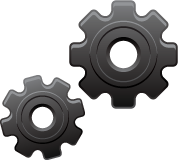# Corrective Mathematics

Efficacy-proven, intensive support for students who have difficulty with mathematics. Corrective Math is organized into seven strategic modules that provide teacher-directed, explicit instruction on critical math skills and concepts students struggle to grasp.Corrective Mathematics is a highly focused, intervention program consisting of seven modules that offer quick, targeting intervention to build understanding of:

• Subtraction
• Multiplication
• Division
• Basic Fractions
• Fractions, Decimals, and Percents
• Ratios and Equations

By focusing on core content and breaking this content into a series of small conceptual steps and embedded skills, Corrective Mathematics enables you to teach mathematics skills, rules, and strategies efficiently and effectively. It ensures that students will learn to work mathematics problems accurately and confidently.

The series is not intended to serve as a fixed course of study. Instead it is used to support a high-quality core mathematics program. A placement test provides a plan that identifies which modules need to be covered.

Student Materials:

• Workbooks include daily worksheets and point summary charts for recording student performance and awarding grades.

Teacher Materials include:

• Presentation Books include a Guide section containing information for presenting exercises, correcting mistakes, and administering the pre-skill and placement tests. There is also a Presentation section that contains detailed lesson plans.
• Answer Key Booklets quickly and easily compare students' work with the actual calculations and word problem results.

• ExamView Software provides dynamic worksheets and creates paper- or computer-based tests and worksheets (available in 2 formats - ExamView for Addition, Subtraction, Multiplication, Division AND ExamView for Basic Fractions; Fractions, Decimals, and Percents; and Ratios and Equations).
• Additional Answer Key Booklets make it easy to check students' independent work.
• Series Guide helps determine what module your students are in by administering the pre-skill and placement tests. Guide also contains sample lessons from each of the seven modules.

## Program Components

•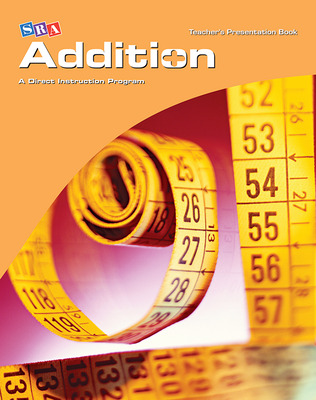•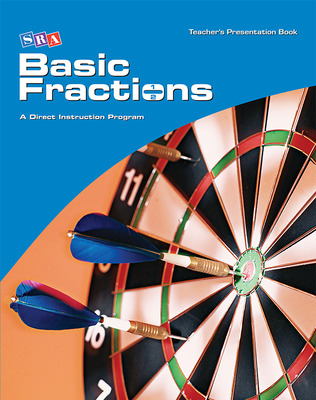Corrective Mathematics Basic Fractions, Teacher Materials
•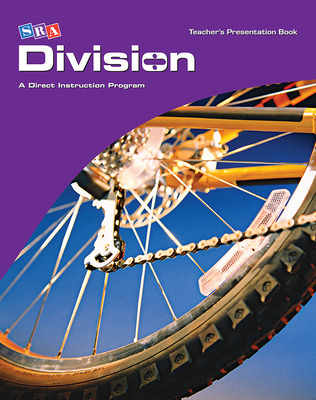Corrective Mathematics Division, Teacher Materials
•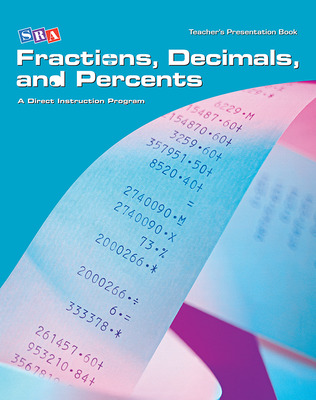Corrective Mathematics Fractions, Decimals, and Percents, Teacher Materials
•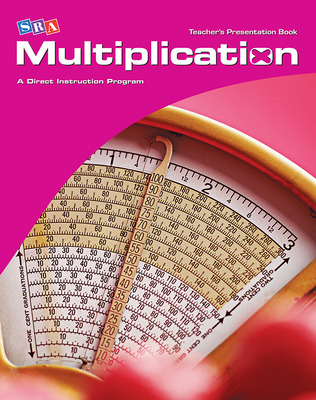Corrective Mathematics Multiplication, Teacher Materials
••Corrective Mathematics Ratios and Equations, Teacher Material
•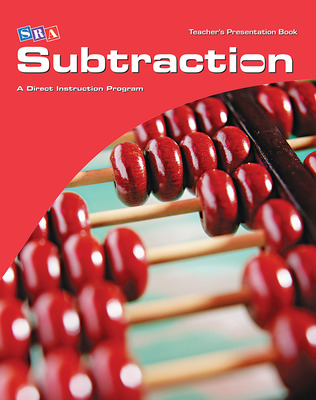Corrective Mathematics Subtraction, Teacher Materials
•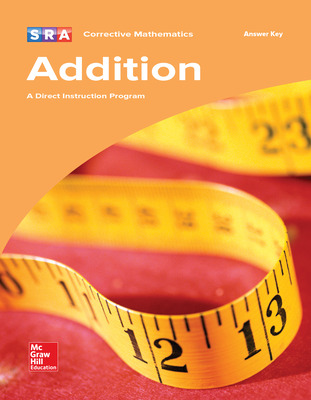•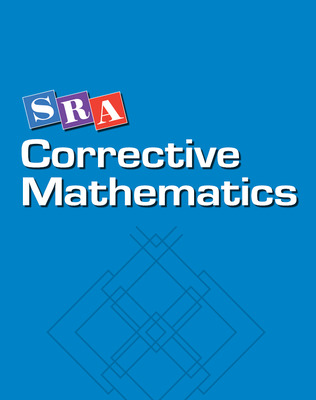Corrective Mathematics Addition, Subtraction, Multiplication, Division, ExamView Local Area Network (LAN) Version
•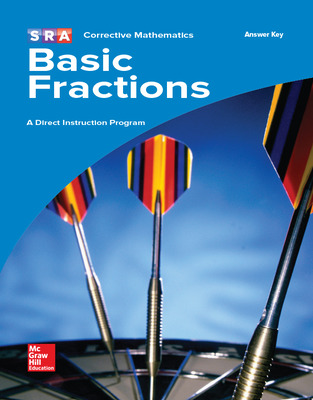•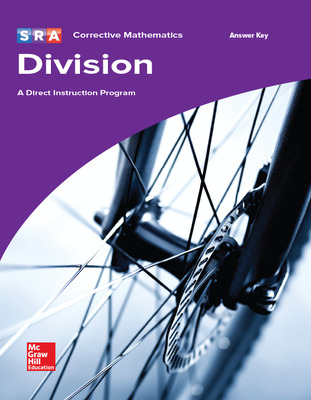•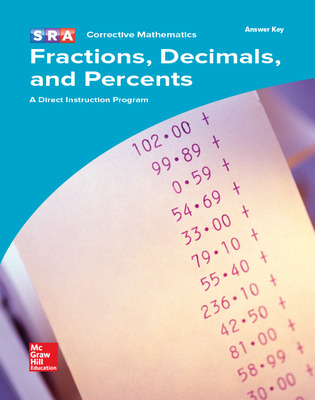•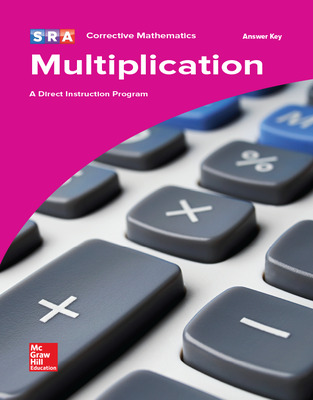•Corrective Mathematics Ratios and Equations, Teacher Presentation Book
•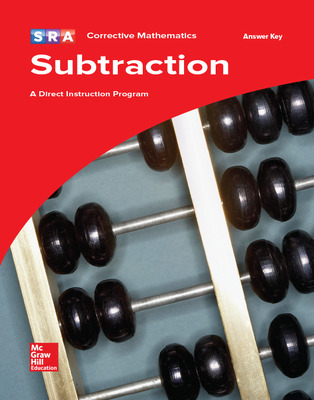•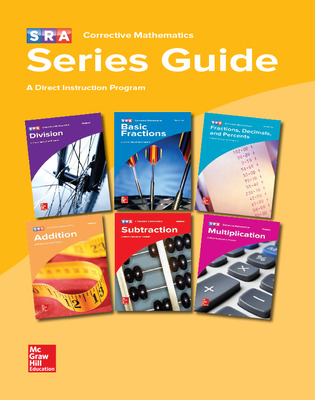Corrective Mathematics, Series Guide
•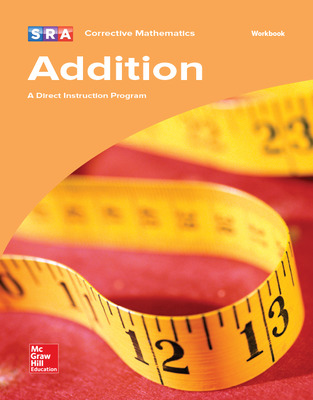•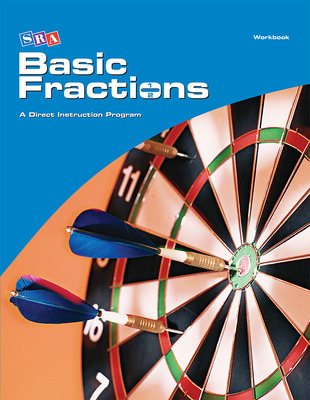Corrective Mathematics Basic Fractions, Workbook
•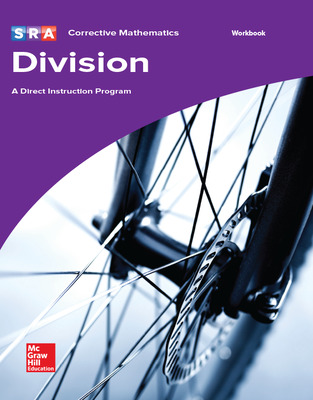Corrective Mathematics Division, Workbook
•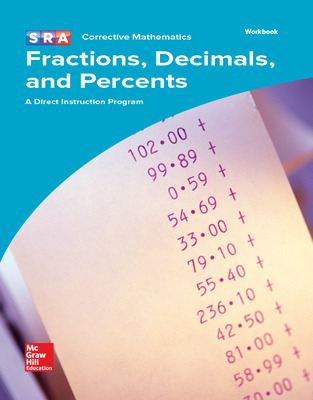Corrective Mathematics Fractions, Decimals, and Percents, Workbook
•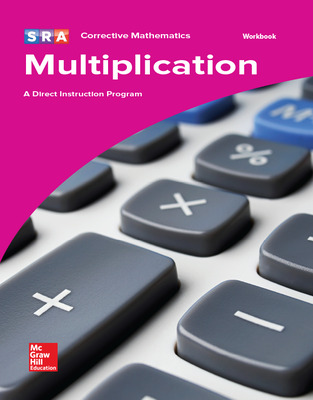Corrective Mathematics Multiplication, Workbook
•Corrective Mathematics Ratios and Equations, Workbook Package of 5
•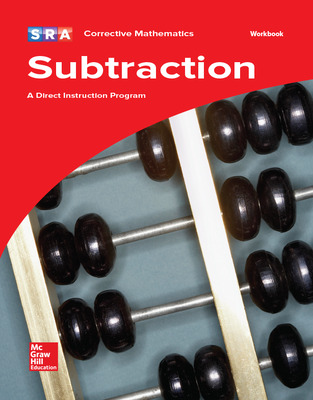Corrective Mathematics Subtraction, Workbook
Sort by:

## Products